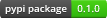# PyBDM: Python interface to the Block Decomposition Method¶The Block Decomposition Method (BDM) approximates algorithmic complexity of a dataset of arbitrary size, that is, the length of the shortest computer program that generates it. This is not trivial as algorithmic complexity is not a computable quantity in the general case and estimation of algorithmic complexity of a dataset can be very useful as it points to mechanistic connections between elements of a system, even such that do not yield any regular statistical patterns that can be captured with more traditional tools based on probability theory and information theory.

Currently 1D and 2D binary arrays are supported, as well as 1D arrays with 4, 5, 6 and 9 discrete symbols.

BDM and the necessary parts of the algorithmic information theory it is based on are described in [STZDG14] and [ZHOK+18].

## Installation¶

Standard installation (stable):

pip install pybdm


Development version installation:

pip install git+https://github.com/sztal/pybdm.git


Local development:

git clone https://github.com/sztal/pybdm
cd pybdm
pip install --editable .


### Supported versions¶

Python3.5+ is supported. Tests are run against Linux, but Windows and OSX should work as well.

## Usage¶

The BDM is implemented using the object-oriented approach and expects input represented as Numpy arrays of integer type.

BDM objects operate exclusively on integer arrays. Hence, any alphabet must be first mapped to a set of integers ranging from 0 to k. Currently only standard numpy arrays are accepted. However, in general it is possible to conceive of a BDM variant optimized for sparse array. We plan provide in the releas.

Detailed description of the design of our implementation of BDM can be found in Theory & Design.

### Binary sequences (1D)¶

import numpy as np
from pybdm import BDM

# Create a dataset (must be of integer type)
X = np.ones((100,), dtype=int)

# Initialize BDM object
# ndim argument specifies dimensionality of BDM
bdm = BDM(ndim=1)

# Compute BDM
bdm.bdm(X)

# BDM objects may also compute standard Shannon entropy in base 2
bdm.ent(X)


### Binary matrices (2D)¶

import numpy as np
from pybdm import BDM

# Create a dataset (must be of integer type)
X = np.ones((100, 100), dtype=int)

# Initialize BDM object
bdm = BDM(ndim=2)

# Compute BDM
bdm.bdm(X)

# BDM objects may also compute standard Shannon entropy in base 2
bdm.ent(X)


### Non-binary sequences (1D)¶

import numpy as np
from pybdm import BDM

# Create a dataset (4 discrete symbols)
np.random.seed(303)
X = np.random.randint(0, 4, (100,))

# Initialize BDM object with 4-symbols alphabet
bdm = BDM(ndim=1, nsymbols=4)

# Compute BDM
bdm.bdm(X)


### Parallel processing¶

PyBDM was designed with parallel processing in mind. Using modern packages for parallelization such as joblib makes it really easy to compute BDM for massive objects.

In this example we will slice a 1000x1000 dataset into 200x200 pieces compute so-called counter objects (final BDM computation operates on such objects) in parallel in 4 independent processes, and aggregate the results into a single BDM approximation of the algorithmic complexity of the dataset.

Remember that data has to be sliced correctly during parallelization in order to ensure fully correct BDM computations. That is, all slices except lower and right boundaries have to be decomposable without any boundary leftovers by the selected decomposition algorithm.

import numpy as np
from joblib import Parallel, delayed
from pybdm import BDM
from pybdm.utils import decompose_dataset

# Create a dataset (must be of integer type)
X = np.ones((1000, 1000), dtype=int)

# Initialize BDM object
bdm = BDM(ndim=2)

# Compute counter objects in parallel
counters = Parallel(n_jobs=4) \
(delayed(bdm.decompose_and_count)(d) for d in decompose_dataset(X, (200, 200)))

# Compute BDM
bdm.compute_bdm(*counters)


### Perturbation analysis¶

Besides the main Block Decomposition Method implementation PyBDM provides also an efficient algorithm for perturbation analysis based on BDM (or standard Shannon entropy).

A perturbation experiment studies change of BDM / entropy under changes applied to the underlying dataset. This is the main tool for detecting parts of a system having some causal significance as opposed to noise parts.

Parts which after yield negative contribution to the overall complexity after change are likely to be important for the system, since their removal make it more noisy. On the other hand parts that yield positive contribution to the overall complexity after change are likely to be noise since they extend the system’s description length.

import numpy as np
from pybdm import BDM
from pybdm.algorithms import PerturbationExperiment

# Create a dataset (must be of integer type)
X = np.ones((100, 100), dtype=int)

# Initialize BDM object
bdm = BDM(ndim=2)

# Initialize perturbation experiment object
# (may be run for both bdm or entropy)
perturbation = PerturbationExperiment(bdm, X, metric='bdm')

# Compute BDM change for all data points
delta_bdm = perturbation.run()

# Compute BDM change for selected data points and keep the changes while running
# One array provide indices of elements that are to be change.
idx = np.array([[0, 0], [10, 10]], dtype=int)
# Another array provide new values to assign.
# Negative values mean that new values will be selected
# randomly from the set of other possible values from the alphabet.
values = np.array([-1, -1], dtype=int)
delta_bdm = perturbation.run(idx, values, keep_changes=True)

# Here is an example applied to an adjacency matrix
# (only 1's are perturbed and switched to 0's)
# (so perturbations correspond to edge deletions)
X = np.random.randint(0, 2, (100, 100))
# Indices of nonzero entries in the matrix
idx = np.argwhere(X)
# PerturbationExperiment can be instantiated without passing data
pe = PerturbationExperiment(bdm, metric='bdm')
# data can be added later
pe.set_data(X)
# Run experiment and perturb edges
# No values argument is passed so perturbations automatically switch
# values to other values from the alphabet (in this case 1 --> 0)
delta_bdm = pe.run(idx)


## Feedback¶

If you have any suggestions or questions about PyBDM feel free to email me at stalaga@protonmail.com.

If you encounter any errors or problems with PyBDM, please let me know! Open an Issue at the GitHub http://github.com/sztal/pybdm main repository.